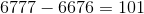# ISEE Middle Level Math : How to find range

## Example Questions

← Previous 1 3 4 5 6 7 8 9 10 11

### Example Question #1 : How To Find Range

On the last day of school,Adam asked each of his classmates how many days of school they missed for the entire school year.  He created a stem-and-leaf plot with the information.

0 | 0 0 2 4 5 6 8

1 | 0 0 1 2

2 | 3

4 | 7

What is the range of the number of days missed by Adam's classmates?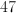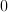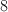Explanation:

To find the range of a set of data, simply subtract the smallest total from the largest total.  The left side of a stem-and-leaf plot represents the ten's digit, and the right side represents the one's digit. The largest value here is 47.  The smallest value is 0.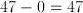### Example Question #3 : Data Analysis And Probability

Find the range in this set of numbers:

4520, 7325, 8732, 8321, 9031, 3117, 6960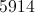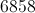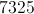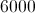Explanation:

First order the numbers from least to greatest: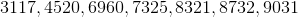Then find the difference of the greatest and the smallest number: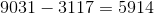### Example Question #4 : Data Analysis And Probability

Find the range in this set of numbers:

6059, 6609, 6906, 6509, 6905, 6960, 6950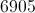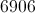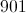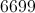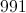Explanation:

First, order the numbers in the set from least to greatest: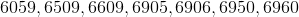Then, find the difference between the largest and the smallest number: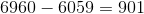### Example Question #5 : Data Analysis And Probability

Find the range in this set of numbers:

640, 782, 411, 398, 299, 344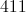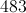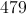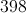Explanation:

First, order the numbers from least to greatest: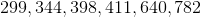Then, find the difference between the largest and smallest number: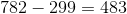### Example Question #6 : Data Analysis And Probability

Find the range in this set of numbers:

9881, 9889, 9818, 9981, 9891, 9918, 9989, 9889, 9198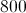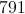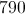Explanation:

First, order the numbers from least to greatest: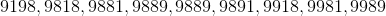Then, find the difference of the largest and smallest number: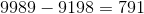### Example Question #7 : Data Analysis And Probability

Find the range in this set of numbers:

3343, 4434, 4334, 3343, 4343, 3434, 3334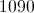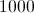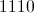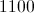Explanation:

First, order the numbers from least to greatest: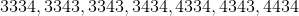Then, find the difference between the largest and the smallest number: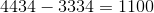### Example Question #8 : Data Analysis And Probability

Subtract the range from the mode in this set of numbers: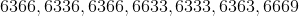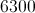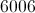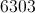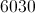Explanation:

First, order the numbers from least to greatest: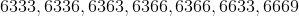Then, identify the mode (most recurring number): 6366

Then, identify the range (subtract the smallest number from the largest):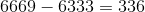Finally, subtract the range from the mode: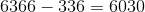### Example Question #1 : How To Find Range

Find the range for this set of numbers:

6363, 6336, 6366, 6633, 6333, 6363, 6663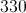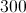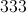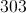Explanation:

First, order the numbers from least to greatest: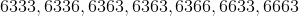Then, find the difference of the largest and the smallest numbers: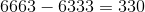### Example Question #1 : How To Find Range

Find the range in this set of numbers:

9080, 9008, 9800, 9099, 9009, 9090, 9008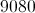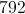Explanation:

First, order the numbers from least to greatest: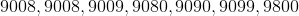The, subtract the smallest number from the greatest: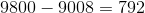### Example Question #1 : How To Find Range

Find the range in this set of numbers: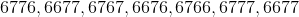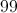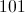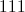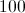Explanation:

First, order the numbers from least to greatest: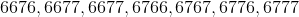Then, find the difference between the largest and smallest number: